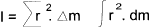Formulae_Index
Nomenclature

Energy Power Formulae

 The standardised acceleration due to gravity ; gn = 9.80665 m /s2 Mass Moment of Inertia The mass moment of inertia of a body about an axis has been defined as the sum of the products of mass-elements and the square of their distance from the axisWork and Power The Work Done W (= Joules = N.m ) by constant Force F_x (N) applied for a distance x (m) W = F_x . x Power is the rate of doing Work P (= Watts = N.m / s ) by constant Force F_x (N) applied for a distance x (m) over t(seconds) P = F_x . x / t also Power = Force F_x at a set velocity v ( N / s) P = F_x . v Rotation The power transmitted by a rotating shaft = the torque T x the angular velocity. P = T * ω   =   T * 2 * π * n P (kW) = T(Nm).n (rev/min) / 9 549 Energy The energy gained by a body during a displacement is equal to the work done by external forces acting upon the body. This includes frictional and non friction forces. The potential energy is the energy possessed by a body by virtue of its position relative to some datum level. The change in potential energy (Joules) gained by a mass of M (kg) lifted through a height of h (metres) ep = M . g n. h The kinetic energy of a a body by virtue of its motion at uniform linear velocity ek = 1/2 . m . v 2 The kinetic energy of a a rotating body ek = 1/2 . I . p 2 Conservation of Energy.. In the absence of any dissipative forces i.e.friction , the sum of the potential energy and kinetic energy remains constant.

Formulae_Index
Nomenclature